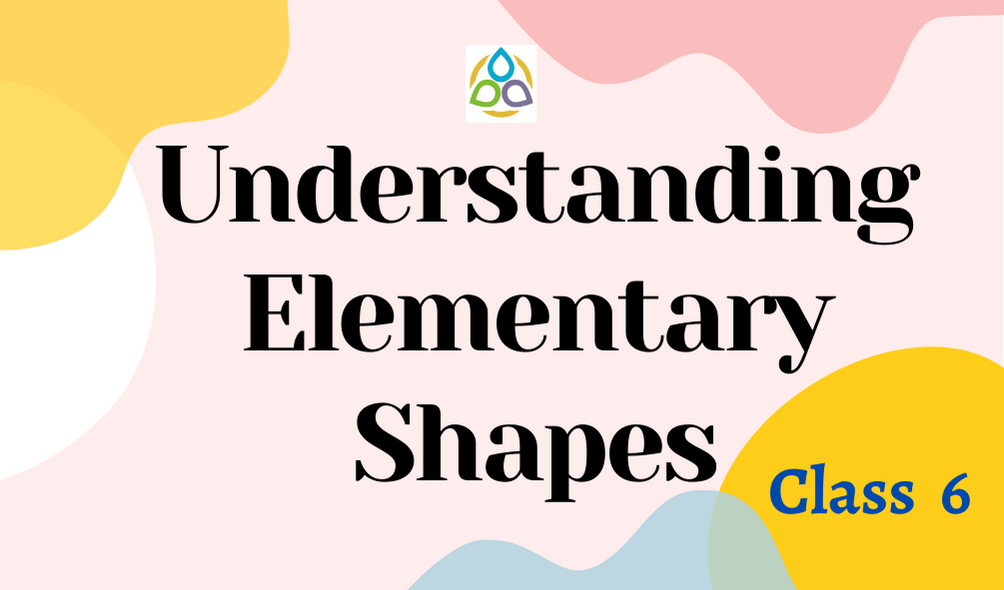# Understanding Elementary Shapes

77. Which of the following is true?

• (A) Length of 1 > Length of 2
• (B) Length of 1 = Length of 2
• (C) Length of 1 < Length of 2
• (D) None of these

76. Which of the following relation is true?

• (A) Measure of a right angle = Measure of a straight angle
• (B) Measure of a right angle = Half the measure of a straight angle
• (C) Measure of a straight angle = Half the measure of a right angle
• (D) None of these

75. Which of the following relation is true?

• (A) Measure of a right angle = Measure of a complete angle
• (B) Measure of a right angle = Half the measure of a complete angle
• (C) Measure of a straight angle = Half the measure of a complete angle
• (D) None of these

74. Half of a revolution of a clock is same as

• (A) 1 right angle
• (B) 2 right angles
• (C) 3 right angles
• (D) None of these

73. One-fourth of a revolution of a clock is same as

• (A) 1 right angle
• (B) 2 right angles
• (C) 3 right angles
• (D) None of these

72. Three-fourths of a revolution of a clock is same as

• (A) 1 right angle
• (B) 2 right angles
• (C) 3 right angles
• (D) None of these

71. What fraction of a clockwise revolution does the hour hand of a clock turn through, when it goes from 12 to 9?

• (A) 1/4
• (B) 1/2
• (C) 3/4
• (D) 2/3

70. Where will the hand of a clock stop if it starts at 12 and makes ½ of a revolution, clockwise?

• (A) 4
• (B) 9
• (C) 6
• (D) 2

69. Where will the hand of a clock stop if it starts at 5 and makes ¾ of a revolution, clockwise?

• (A) 4
• (B) 1
• (C) 3
• (D) 2

68. Where will the hand of a clock stop if it starts at 7 and makes ¼ of a revolution, anti-clockwise?

• (A) 4
• (B) 1
• (C) 3
• (D) 2

67. What part of a revolution have you turned through if you stand facing east and turn clockwise to face north?

• (A) 3/4
• (B) 1/4
• (C) 1/2
• (D) 2/3

66. What part of a revolution have you turned through if you stand facing west and turn ant-clockwise to face east?

• (A) 3/4
• (B) 1/4
• (C) 1/2
• (D) 2/3

65. Where will the hour hand of a clock stop if it starts from 8 and turns through 2 right angles?

• (A) 3
• (B) 1
• (C) 4
• (D) 2

64. Where will the hour hand of a clock stop if it starts from 10 and turns through 3 right angles?

• (A) 3
• (B) 4
• (C) 6
• (D) 7

63. What is the difference between 180° and 157½°?

• (A) 33½°
• (B) 32½°
• (C) 23½°
• (D) 22½°